# ML Aggarwal Class 7 Solutions for ICSE Maths Chapter 8 Algebraic Expressions Ex 8.3

## ML Aggarwal Class 7 Solutions for ICSE Maths Chapter 8 Algebraic Expressions Ex 8.3

Question 1.
If m = 2, find the value of:
(i) 3m – 5
(ii) 9 – 5m
(iii) 3m2 – 2m – 1
(iv) $$\frac { 5 }{ 2 }$$ m – 4
Solution: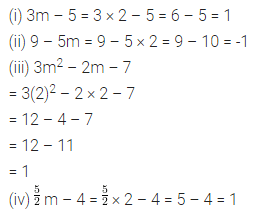Question 2.
If p = -2, find the value of:
(1) 4p + 7
(ii) -3p2 + 4p + 7
(iii) -2p3 – 3p2 + 4p + 7
Solution:Question 3.
If a = 2, b = -2, find the value of:
(i) a2 + b2
(ii) a2 + ab + b2
(iii) a2 – b2
Solution:Question 4.
When a = 0, b = -1, find the value of the given expressions:
(i) 2a2 + b2 + 1
(ii) a2 + ab + 2
(iii) 2a2b + 2ab2 + ab
Solution: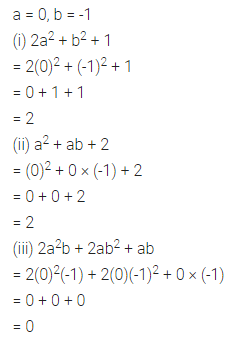Question 5.
If p = -10, find the value of p2 – 2p – 100.
Solution:Question 6.
If z = 10, find the value of z3 – 3(z – 10).
Solution: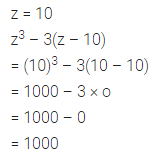Question 7.
Simplify the following expressions and find their values when x = 2:
(i) x + 7 + 4(x – 5)
(ii) 3(x + 2) + 5x – 7
(iii) 6x + 5(x – 2)
(iv) 4(2x – 1) + 3x + 11
Solution: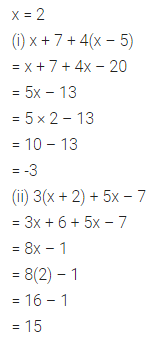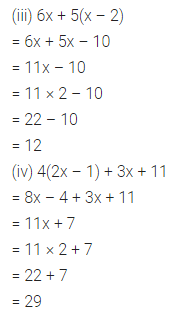Question 8.
Simplify the following expressions and find their values when a = -1, b = -2:
(i) 2a – 2b – 4 – 5 + a
(ii) 2(a2 + ab) + 3 – ab
Solution: